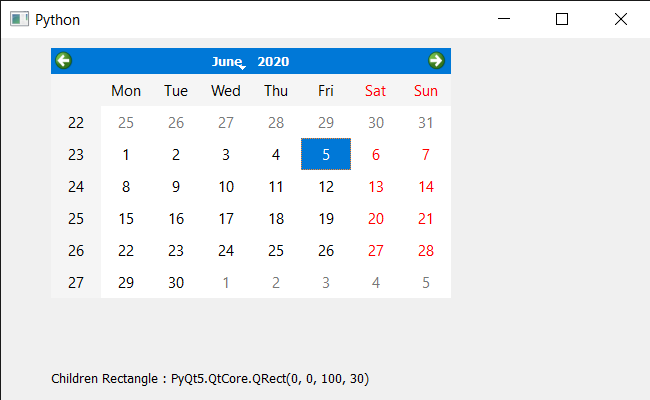Related Articles

# PyQt5 QCalendarWidget – Accessing Children Rectangle

• Last Updated : 08 Jun, 2020

In this article we will see how we get the children rectangle of the QCalendarWidget. Calendar is not alone a single widget it is a mixture of lots of smaller widget which we refer as calendar’s child. There are many child such as table view, item delegate etc, we use `children` method to get all the children of it. Children rectangle is the bounding rectangle of all the children except hidden children.

In order to do this we will use `childrenRect` method with the QCalendarWidget object.

Syntax : calendar.childrenRect()

Argument : It takes no argument

Return : It return QRect object

Below is the implementation

 `# importing libraries``from` `PyQt5.QtWidgets ``import` `*` `from` `PyQt5 ``import` `QtCore, QtGui``from` `PyQt5.QtGui ``import` `*` `from` `PyQt5.QtCore ``import` `*` `import` `sys`` ` ` ` `class` `Window(QMainWindow):`` ` `    ``def` `__init__(``self``):``        ``super``().__init__()`` ` `        ``# setting title``        ``self``.setWindowTitle(``"Python "``)`` ` `        ``# setting geometry``        ``self``.setGeometry(``100``, ``100``, ``650``, ``400``)`` ` `        ``# calling method``        ``self``.UiComponents()`` ` `        ``# showing all the widgets``        ``self``.show()`` ` `    ``# method for components``    ``def` `UiComponents(``self``):`` ` `        ``# creating a QCalendarWidget object``        ``self``.calender ``=` `QCalendarWidget(``self``)`` ` `        ``# setting geometry to the calender``        ``self``.calender.setGeometry(``50``, ``10``, ``400``, ``250``)`` ` `        ``# creating a label``        ``label ``=` `QLabel(``self``)`` ` `        ``# setting geometry``        ``label.setGeometry(``50``, ``280``, ``420``, ``120``)`` ` `        ``# making it multi line``        ``label.setWordWrap(``True``)`` ` `        ``# getting children rectangle ``        ``value ``=` `self``.calender.childrenRect()`` ` `        ``# setting text to the label``        ``label.setText(``"Children Rectangle : "` `+` `str``(value))`` ` ` ` ` ` `# create pyqt5 app``App ``=` `QApplication(sys.argv)`` ` `# create the instance of our Window``window ``=` `Window()`` ` ` ` `# start the app``sys.exit(App.``exec``())`

Output :Attention geek! Strengthen your foundations with the Python Programming Foundation Course and learn the basics.

To begin with, your interview preparations Enhance your Data Structures concepts with the Python DS Course. And to begin with your Machine Learning Journey, join the Machine Learning – Basic Level Course

My Personal Notes arrow_drop_up